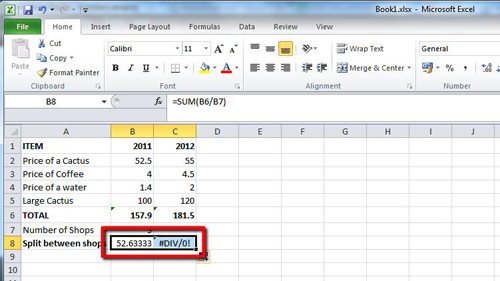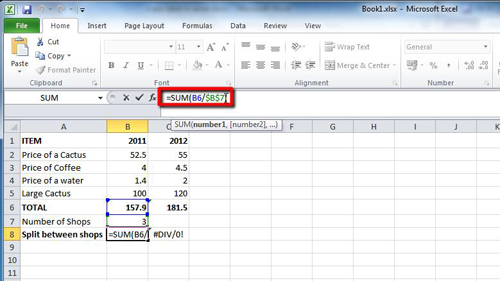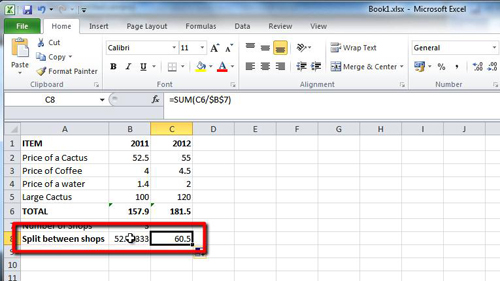## How to Use Excel 2010 Absolute Reference

The default setting in Excel is to have relative formulas, but sometimes making them have absolute references is useful – this tutorial is going to show you how to use excel absolute reference for formulas.

Step # 1 – Identifying the Formula for being made Absolute

Start Excel with the sheet where you need to create an absolute reference. In this example you can see that we are dividing the “TOTAL” by the “Number of Shops” to give the “Split between shops”. This is done with a simple SUM formula, which you can see in cell “C8”. This is currently a relative formula and if we move it to cell “C8” to calculate the split for 2012 it will not work.Step # 2 – Making Part of the Formula Absolute

This formula needs to be made absolute so it will always reference the value in cell “B7”. Click on the cell, “B8” in this case, to edit the formula. To make the “B7” value absolute, delete “B7” and type “\$B\$7” – dollar signs in these locations make the value absolute.Step # 3 – Completing the Calculation with the Absolute Reference

Press return to complete the change to the formula. Now when you try and copy the formula to the adjacent cell there will not be an error and the “TOTAL” will be divided by 3. This is how to use excel absolute reference and you can see it successfully gives us a result of “60.5”